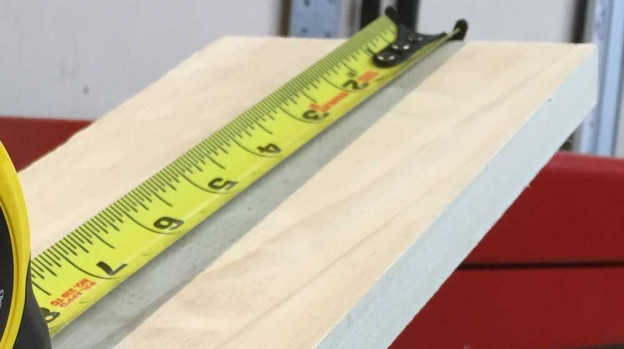# How to Calculate Linear FeetLinear footage might not be a familiar measurement to you, and you might be asking yourself what it is and how to find it, or it could simply be a different name for something you are already familiar with. Either way, keep reading to find out!

## What is a Linear Foot?

A linear foot is simply a length measurement equal to one foot. To find linear footage, you simply need to measure the length in feet, that’s it.

Linear footage is simply another name for a length or distance measured in feet.

The term “linear” means a shape or movement in a straight line. So, a linear foot measurement is a straight line measurement. For example, width, height, and thickness measurements in feet are all examples of linear foot measurements.

Linear footage is a measure of length and is commonly used to measure an object or material’s length. You might also see this measurement referred to as a lineal foot.

Linear feet and the length in feet are the same measurements, so one foot is equal to one linear foot.

You might see some supplies sold using this measurement. For example, flooring, lumber, fencing, and fabrics are commonly sold by the linear foot.

## How to Find Linear Footage

Finding linear footage requires measuring a length in feet. Use a tape measure and measure the length of your space or object.

Since linear footage is a one-dimensional measurement of length, measurements of width or thickness are not necessary unless you want to calculate an area or volume.

If your measurement is not in feet, simply convert to feet, and you have the correct measurement. For example, use our inch fraction calculator to convert inches or centimeters to feet.

## How to Find the Linear Feet of Lumber Boards

To find the total length in linear feet of multiple pieces of lumber, you need to measure the length of each piece and add the measurements together.

Take note of each board’s length, and after all measurements are complete, then use a calculator such as our feet and inches calculator to add the feet measurements together.

## How to Calculate Linear Footage Price

Calculating the cost of lumber priced by the linear foot requires finding the total length in feet and then multiplying by the price per foot. Thus, the total cost is equal to the total length in feet times the price per foot.

When ordering material this way, it’s a good idea to consider the typical board lengths of lumber that are sold since you may need additional material to avoid unnecessarily joining boards together. As a general rule of thumb, we recommend ordering an extra 10% of materials to account for off-cuts and waste as a general rule of thumb.

## How are Linear Feet Different From Square Feet?

You might be wondering how linear feet are different from square feet.

Recall that linear footage is a one-dimensional length measurement. On the other hand, square footage is an area measurement, and thus is a two-dimensional measurement.

You can use our conversion calculator to calculate square feet from linear feet measurements and vice-versa.

## Common Lengths in Linear Feet

Refer to the chart below for common lengths in inches and their equivalent linear footage measurements.

Linear feet measurements for common inch measurements in 6″ increments.
Distance in Inches Linear Feet
6″ 0.5 ft
12″ 1 ft
18″ 1.5 ft
24″ 2 ft
30″ 2.5 ft
36″ 3 ft
42″ 3.5 ft
48″ 4 ft
54″ 4.5 ft
60″ 5 ft
66″ 5.5 ft
72″ 6 ft
78″ 6.5 ft
84″ 7 ft
90″ 7.5 ft
96″ 8 ft
102″ 8.5 ft
108″ 9 ft
114″ 9.5 ft
120″ 10 ft
126″ 10.5 ft
132″ 11 ft
138″ 11.5 ft
144″ 12 ft
150″ 12.5 ft
156″ 13 ft
162″ 13.5 ft
168″ 14 ft
174″ 14.5 ft
180″ 15 ft
186″ 15.5 ft
192″ 16 ft
198″ 16.5 ft
204″ 17 ft
210″ 17.5 ft
216″ 18 ft
222″ 18.5 ft
228″ 19 ft
234″ 19.5 ft
240″ 20 ft

## References

1. Collins Dictionary, linear, https://www.collinsdictionary.com/us/dictionary/english/linear